# Discrete Mathematics and Combinatorics Commons™

1,115 Full-Text Articles 1,295 Authors 292,068 Downloads114 Institutions

## All Articles in Discrete Mathematics and Combinatorics

1,115 full-text articles. Page 5 of 44.

Distribution Of The P-Torsion Of Jacobian Groups Of Regular Matroids, 2021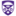The University of Western Ontairo

#### Distribution Of The P-Torsion Of Jacobian Groups Of Regular Matroids, Sergio R. Zapata Ceballos

##### Electronic Thesis and Dissertation Repository

Given a regular matroid $M$ and a map $\lambda\colon E(M)\to \N$, we construct a regular matroid $M_\lambda$. Then we study the distribution of the $p$-torsion of the Jacobian groups of the family $\{M_\lambda\}_{\lambda\in\N^{E(M)}}$. We approach the problem by parameterizing the Jacobian groups of this family with non-trivial $p$-torsion by the $\F_p$-rational points of the configuration hypersurface associated to $M$. In this way, we reduce the problem to counting points over finite fields. As a result, we obtain a closed formula for the proportion of these groups with non-trivial $p$-torsion as well as some estimates. In addition, we show that the …

Introduction To Discrete Mathematics: An Oer For Ma-471, 2021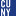CUNY Queensborough Community College

#### Introduction To Discrete Mathematics: An Oer For Ma-471, Mathieu Sassolas

##### Open Educational Resources

The first objective of this book is to define and discuss the meaning of truth in mathematics. We explore logics, both propositional and first-order , and the construction of proofs, both formally and human-targeted. Using the proof tools, this book then explores some very fundamental definitions of mathematics through set theory. This theory is then put in practice in several applications. The particular (but quite widespread) case of equivalence and order relations is studied with detail. Then we introduces sequences and proofs by induction, followed by number theory. Finally, a small introduction to combinatorics is …

Upper Bounds For Inverse Domination In Graphs, 2021Clayton State University

#### Upper Bounds For Inverse Domination In Graphs, Elliot Krop, Jessica Mcdonald, Gregory J. Puleo

##### Theory and Applications of Graphs

In any graph $G$, the domination number $\gamma(G)$ is at most the independence number $\alpha(G)$. The \emph{Inverse Domination Conjecture} says that, in any isolate-free $G$, there exists pair of vertex-disjoint dominating sets $D, D'$ with $|D|=\gamma(G)$ and $|D'| \leq \alpha(G)$. Here we prove that this statement is true if the upper bound $\alpha(G)$ is replaced by $\frac{3}{2}\alpha(G) - 1$ (and $G$ is not a clique). We also prove that the conjecture holds whenever $\gamma(G)\leq 5$ or $|V(G)|\leq 16$.

Skolem Number Of Cycles And Grid Graphs, 2021Southern Connecticut State University

#### Skolem Number Of Cycles And Grid Graphs, Braxton Carrigan, John Asplund

##### Theory and Applications of Graphs

A Skolem sequence can be thought of as a labelled path where two vertices with the same label are that distance apart. This concept has naturally been generalized to labellings of other graphs, but always using at most two of any integer label. Given that more than two vertices can be mutually distance d apart, we define a new generalization of a Skolem sequences on graphs that we call proper Skolem labellings. This brings rise to the question; what is the smallest set of consecutive positive integers we can use to proper Skolem label a graph?'' This will be known …

Domino Circles, 2021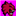Bard College

#### Domino Circles, Lauren L. Rose, A. Gwinn Royal, Amanda Serenevy, Anna Varvak

##### Journal of Math Circles

Creating a circle with domino pieces has a connection with complete graphs in Graph Theory. We present a hands-on activity for all ages, using dominoes to explore problem solving, pattern recognition, parity, graph theory, and combinatorics. The activities are suitable for elementary school students, the graph theory interpretations are suitable for middle and high school students, and the underlying mathematical structures will be of interest to college students and beyond.

An Upper Bound On The Spectral P-Norms Of Tensors And Matrix Permanent, 2021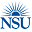Nova Southeastern University

#### An Upper Bound On The Spectral P-Norms Of Tensors And Matrix Permanent, Killian J. Hitsman, Vehbi E. Paksoy

##### Mako: NSU Undergraduate Student Journal

No abstract provided.

Categorical Aspects Of Graphs, 2021Western University

#### Categorical Aspects Of Graphs, Jacob D. Ender

##### Undergraduate Student Research Internships Conference

In this article, we introduce a categorical characterization of directed and undirected graphs, and explore subcategories of reflexive and simple graphs. We show that there are a number of adjunctions between such subcategories, exploring varying combinations of graph types.

Spectral Graph Theory And Research, 2021Western University

#### Spectral Graph Theory And Research, Nathan H. Kershaw, Lewis Glabush

##### Undergraduate Student Research Internships Conference

Our topic of study was Spectral Graph Theory. We studied the algebraic methods used to study the properties of graphs (networks) and became familiar with the applications of network analysis. We spent a significant amount of time studying the way virus’s spread on networks, with particular applications to Covid-19. We also investigated the relationship between graph spectra and structural properties.

On \Delta^(K)-Colouring Of Powers Of Paths And Cycles, 2021Christ University, Bangalore

#### On \Delta^(K)-Colouring Of Powers Of Paths And Cycles, Merlin Thomas Ellumkalayil Ms, Sudev Naduvath

##### Theory and Applications of Graphs

In a proper vertex colouring of a graph, the vertices are coloured in such a way that no two adjacent vertices receive the same colour, whereas in an improper vertex colouring, adjacent vertices are permitted to receive same colours subjected to some conditions. An edge of an improperly coloured graph is said to be a bad edge if its end vertices have the same colour. A near proper colouring is a colouring which minimises the number of bad edges by restricting the number of colour classes that can have adjacency among their own elements. The $\delta^{(k)}$- colouring is a near …

On Graphs With Proper Connection Number 2, 2021University of Alaska Fairbanks

#### On Graphs With Proper Connection Number 2, Jill Faudree, Leah Berman, Glenn Chappell, Chris Hartman, John Gimbel, Gordon Williams

##### Theory and Applications of Graphs

An edge-colored graph is properly connected if for every pair of vertices u and v there exists a properly colored uv-path (i.e. a uv-path in which no two consecutive edges have the same color). The proper connection number of a connected graph G, denoted pc(G), is the smallest number of colors needed to color the edges of G such that the resulting colored graph is properly connected. An edge-colored graph is flexibly connected if for every pair of vertices u and v there exist two properly colored paths between them, say P and Q, such that the first edges of …

2021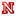University of Nebraska-Lincoln

#### A Combinatorial Formula For Kazhdan-Lusztig Polynomials Of Sparse Paving Matroids, George Nasr

##### Dissertations, Theses, and Student Research Papers in Mathematics

We present a combinatorial formula using skew Young tableaux for the coefficients of Kazhdan-Lusztig polynomials for sparse paving matroids. These matroids are known to be logarithmically almost all matroids, but are conjectured to be almost all matroids. We also show the positivity of these coefficients using our formula. In special cases, such as for uniform matroids, our formula has a nice combinatorial interpretation.

Bootstrap Percolation On Random Geometric Graphs, 2021University of Nebraska - Lincoln

#### Bootstrap Percolation On Random Geometric Graphs, Alyssa Whittemore

##### Dissertations, Theses, and Student Research Papers in Mathematics

Bootstrap Percolation is a discrete-time process that models the spread of information or disease across the vertex set of a graph. We consider the following version of this process:

Initially, each vertex of the graph is set active with probability p or inactive otherwise. Then, at each time step, every inactive vertex with at least k active neighbors becomes active. Active vertices will always remain active. The process ends when it reaches a stationary state. If all the vertices eventually become active, then we say we achieve percolation.

This process has been widely studied on many families of graphs, deterministic …

Partially Oriented 6-Star Decomposition Of Some Complete Mixed Graphs, 2021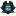East Tennessee State University

#### Partially Oriented 6-Star Decomposition Of Some Complete Mixed Graphs, Kazeem A. Kosebinu

##### Electronic Theses and Dissertations

Let $M_v$ denotes a complete mixed graph on $v$ vertices, and let $S_6^i$ denotes the partial orientation of the 6-star with twice as many arcs as edges. In this work, we state and prove the necessary and sufficient conditions for the existence of $\lambda$-fold decomposition of a complete mixed graph into $S_6^i$ for $i\in\{1,2,3,4\}$. We used the difference method for our proof in some cases. We also give some general sufficient conditions for the existence of $S_6^i$-decomposition of the complete bipartite mixed graph for $i\in\{1,2,3,4\}$. Finally, this work introduces the decomposition of a complete mixed graph with a hole into …

Partial Representations For Ternary Matroids, 2021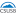California State University, San Bernardino

#### Partial Representations For Ternary Matroids, Ebony Perez

##### Electronic Theses, Projects, and Dissertations

In combinatorics, a matroid is a discrete object that generalizes various notions of dependence that arise throughout mathematics. All of the information about some matroids can be encoded (or represented) by a matrix whose entries come from a particular field, while other matroids cannot be represented in this way. However, for any matroid, there exists a matrix, called a partial representation of the matroid, that encodes some of the information about the matroid. In fact, a given matroid usually has many different partial representations, each providing different pieces of information about the matroid. In this thesis, we investigate when a …

The Color Number Of Cubic Graphs Having A Spanning Tree With A Bounded Number Of Leaves, 2021Mindanao State University - Iligan Institute of Technology

#### The Color Number Of Cubic Graphs Having A Spanning Tree With A Bounded Number Of Leaves, Analen A. Malnegro, Gina A. Malacas, Kenta Ozeki

##### Theory and Applications of Graphs

The color number c(G) of a cubic graph G is the minimum cardinality of a color class of a proper 4-edge-coloring of G. It is well-known that every cubic graph G satisfies c(G) = 0 if G has a Hamiltonian cycle, and c(G) ≤ 2 if G has a Hamiltonian path. In this paper, we extend these observations by obtaining a bound for the color number of cubic graphs having a spanning tree with a bounded number of leaves.

2021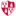Montclair State University

#### Contributions To The Teaching And Learning Of Fluid Mechanics, Ashwin Vaidya

##### Department of Mathematics Facuty Scholarship and Creative Works

This issue showcases a compilation of papers on fluid mechanics (FM) education, covering different sub topics of the subject. The success of the first volume  prompted us to consider another follow-up special issue on the topic, which has also been very successful in garnering an impressive variety of submissions. As a classical branch of science, the beauty and complexity of fluid dynamics cannot be overemphasized. This is an extremely well-studied subject which has now become a significant component of several major scientific disciplines ranging from aerospace engineering, astrophysics, atmospheric science (including climate modeling), biological and biomedical science …

On Communication For Distributed Babai Point Computation, 2021Simula UiB

#### On Communication For Distributed Babai Point Computation, Maiara F. Bollauf, Vinay A. Vaishampayan, Sueli I.R. Costa

##### Publications and Research

We present a communication-efficient distributed protocol for computing the Babai point, an approximate nearest point for a random vector X∈Rn in a given lattice. We show that the protocol is optimal in the sense that it minimizes the sum rate when the components of X are mutually independent. We then investigate the error probability, i.e. the probability that the Babai point does not coincide with the nearest lattice point, motivated by the fact that for some cases, a distributed algorithm for finding the Babai point is sufficient for finding the nearest lattice point itself. Two different probability models for X …

Irreducibility And Galois Groups Of Random Polynomials, 2021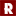Stanford University

#### Irreducibility And Galois Groups Of Random Polynomials, Hanson Hao, Eli Navarro, Henri Stern

In 2015, I. Rivin introduced an effective method to bound the number of irreducible integral polynomials with fixed degree d and height at most N. In this paper, we give a brief summary of this result and discuss the precision of Rivin's arguments for special classes of polynomials. We also give elementary proofs of classic results on Galois groups of cubic trinomials.

Repeat Length Of Patterns On Weaving Products, 2021Rose-Hulman Institute of Technology

#### Repeat Length Of Patterns On Weaving Products, Zhuochen Liu

On weaving products such as fabrics and silk, people use interlacing strands to create artistic patterns. Repeated patterns form aesthetically pleasing products. This research is a mathematical modeling of weaving products in the real world by using cellular automata. The research is conducted by observing the evolution of the model to better understand products in the real world. Specifically, this research focuses on the repeat length of a weaving pattern given the rule of generating it and the configuration of the starting row. Previous studies have shown the range of the repeat length in specific situations. This paper will generalize …

New Results On Subtractive Magic Graphs, 2021Centre College

#### New Results On Subtractive Magic Graphs, Matthew J. Ko, Jason Pinto, Aaron Davis